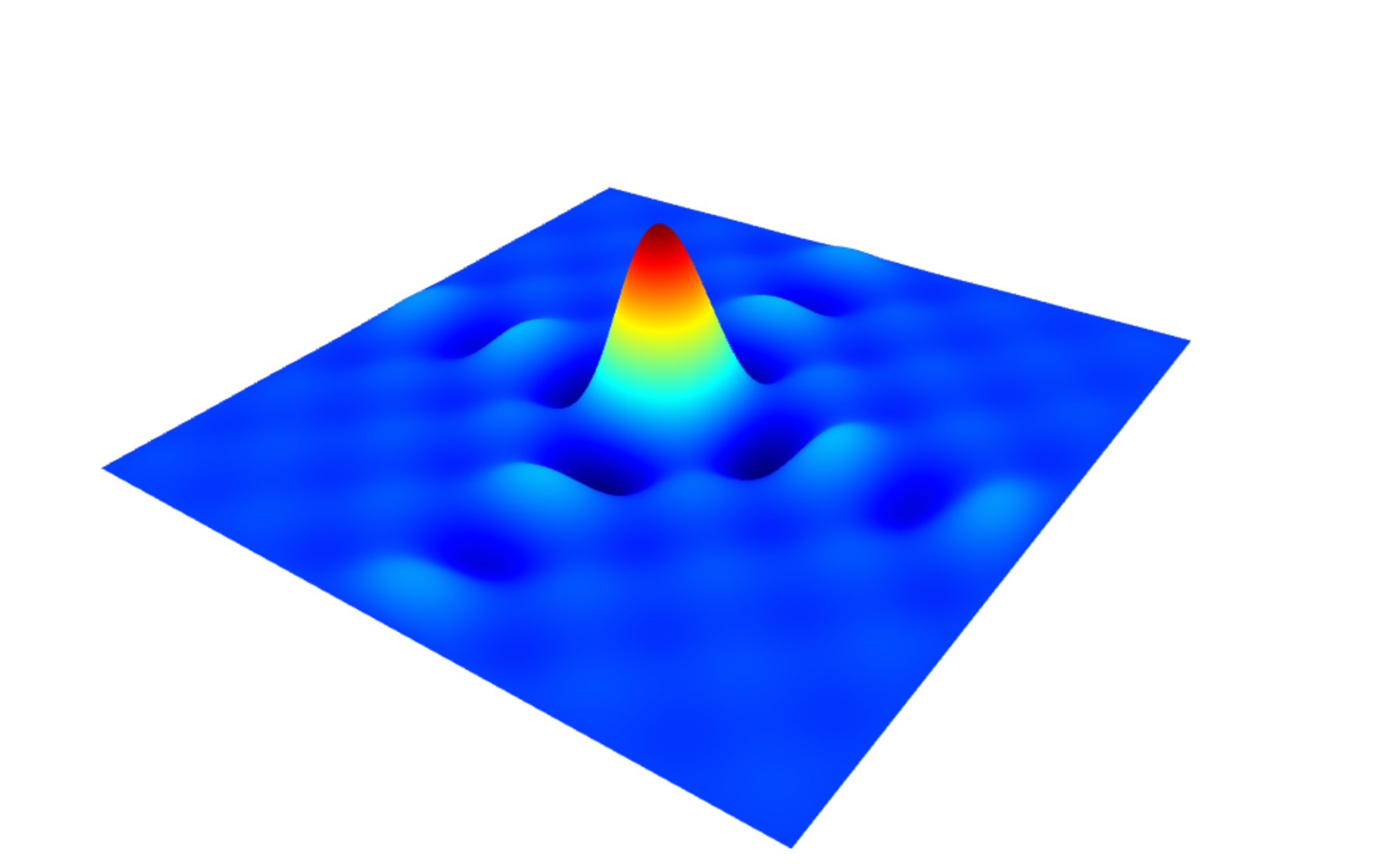# gl-surface3d

Renders parametric surfaces in 3D

## Usage no npm install needed!

``````<script type="module">
import glSurface3d from 'https://cdn.skypack.dev/gl-surface3d';
</script>``````

# gl-surface3d

3D parametric surface plotting, compatible with gl-vis.

# Example

``````var createScene       = require('gl-plot3d')
var createSurfacePlot = require('gl-surface3d')
var ndarray           = require('ndarray')
var fill              = require('ndarray-fill')
var diric             = require('dirichlet')

var scene = createScene()

//Create field
var field = ndarray(new Float32Array(512*512), [512,512])
fill(field, function(x,y) {
return 128 * diric(10, 10.0*(x-256)/512) * diric(10, 10.0*(y-256)/512)
})

//Create surface plot
var surface = createSurfacePlot({
gl:    scene.gl,
field: field
})

``````

Here is what this should look like:Test it in your browser (requires WebGL)

# Install

``````npm install gl-surface3d
``````

# Basic Interface

## Constructor

#### `var surface = require('gl-surface3d')(params)`

Creates a surface plot object. `params` is an object with any of the following parameters

• `gl` is a WebGL context
• `field` a new 2D field encoded as an ndarray
• `coords` is an array of 3 2D fields, each encoded as ndarrays (for parameteric surfaces)
• `intensity` a 2D intensity field (defaults to `field` or `coords is not present)
• `colormap` the name of the new color map for the surface (see list of names in `colormap` docs)
• `intensityBounds` sets the intensity range for the colormap
• `ticks` is a pair of arrays of ticks representing the spacing of the points for the axes of the surface
• `showSurface` if set, draw the surface
• `showContour` if set, draw contour lines
• `contourWidth` the width fo the contour lines
• `contourTint` the amount of tint of the contour lines
• `contourColor` the color of the contour line tint
• `levels` an array of arrays representing the level of the isolines.
• `dynamicWidth` the width of the dynamic isolines
• `dynamicColors` the color of the dynamic isolines
• `dynamicTint` the tint of the dynamic isolines
• `vertexColor` interpolate color in fragment or vertex shader, useful for discontinuous functions (ref)

## Methods

#### `surface.update(params)`

Modify the surface. `params` is an object with the same properties as the constructor